3D Quiver Plot Helper VI

LabVIEW 2018 Help

Edition Date: March 2018
Part Number: 371361R-01
View Product InfoLabVIEW 2016 HelpLabVIEW 2017 HelpLabVIEW 2018 HelpLabVIEW 2019 HelpLabVIEW 2020 Help

Requires: Full Development System

Graphs a plot of normal vectors. Multiple references to this VI generate multiple plots for the same graph.Note  This VI appears on the block diagram when you add a Quiver plot to the front panel. You can find the Quiver plot on the 3D Graph palette.

Example

Use the pull-down menu to select an instance of this VI.

 Select an instance VectorMatrix

Vector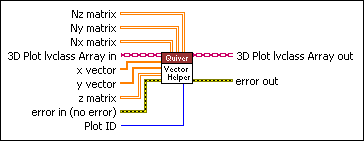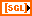Nz matrix is a 2D array that specifies the length of the z vector in relation to the z-plane.Ny matrix is a 2D array that specifies the length of the y vector in relation to the z-plane.Nx matrix is a 2D array that specifies the length of the x vector in relation to the z-plane.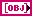3D Plot lvclass Array in is a reference to the class that stores the data of the 3D plot.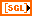x vector is a 1D array that specifies the x-coordinates that graph the plot.y vector is a 1D array that specifies the y-coordinates that graph the plot.z matrix is a 2D array that specifies the z-coordinates that graph the plot. If you do not wire the other inputs, LabVIEW graphs the number of elements for the x-axis based on the number of rows in z matrix and the number of elements for the y-axis based on the number of columns in z matrix.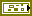error in describes error conditions that occur before this node runs. This input provides standard error in functionality.Plot ID specifies the index of the plot you want to graph. When you graph more than one plot, select the drop-down listbox under the color spectrum to the right of the graph to change the plot.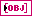3D Plot lvclass Array out is the graph of the 3D plot.error out contains error information. This output provides standard error out functionality.

Matrix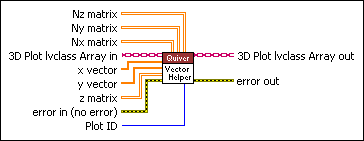Nz matrix is a 2D array that specifies the length of the z vector in relation to the z-plane.Ny matrix is a 2D array that specifies the length of the y vector in relation to the z-plane.Nx matrix is a 2D array that specifies the length of the x vector in relation to the z-plane.3D Plot lvclass Array in is a reference to the class that stores the data of the 3D plot.x matrix is a 2D array that specifies the x-coordinates that graph the plot.y matrix is a 2D array that specifies the y-coordinates that graph the plot.z matrix is a 2D array that specifies the z-coordinates that graph the plot. If you do not wire the other inputs, LabVIEW graphs the number of elements for the x-axis based on the number of rows in z matrix and the number of elements for the y-axis based on the number of columns in z matrix.error in describes error conditions that occur before this node runs. This input provides standard error in functionality.Plot ID specifies the index of the plot you want to graph. When you graph more than one plot, select the drop-down listbox under the color spectrum to the right of the graph to change the plot.3D Plot lvclass Array out is the graph of the 3D plot.error out contains error information. This output provides standard error out functionality.

Example

Refer to the 3D Quiver Graph VI in the labview\examples\Controls and Indicators\Graphs and Charts\Math Plots - 3D directory for an example of using the 3D Quiver Plot Helper VI.